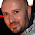Hi! Hope you're enjoying this blog. I have a new home at www.goldsborough.me. Be sure to also check by there for new posts <3

## Sunday, July 20, 2014

### Creating complex waveforms through Fourier / Additive Synthesis - Version 2

In the last post in my "Developing a digital synthesizer in C++" series, I described how Fourier Synthesis, also known as Additive synthesis, could be used to create complex waveforms of any shape or form. In the recent months I have continued to work on my synthesizer and progressed a lot with my knowledge, therefore I have revised and improved lots of my code, including my functions for creating complex waveforms. Because the source code I provided in the last post has lots of problems, I decided to publish my revised code. I will only explain why and how I changed the previous code, so I recommend you have a look at the previous post if you haven't yet, especially if you want to learn about Fourier synthesis in general.

The main problem with the previous code was that it was not generic at all and actually didn't follow one of the basic rules about functions: they should do one thing and one thing only. The function might be good enough if you want to create only a handful of different waveforms, say square, sine and saw, but as soon as we're talking about creating any waveform we want to, using the same function, we'd be getting into problems pretty soon. The solution I found to this problem is quite nice, but it requires a few more initial steps.

In Fourier Synthesis, a partial is generally described by its frequency, its amplitude and optionally its phase offset. Because a partial is an integer multiple of a fundamental frequency and given the fact that wavetables usually store waveforms with a period of exactly 1 Hertz, the frequency is directly related to the number of the partial, so that's all we need.

Now that we know what information we need about each partial, we can create such a struct:

```struct Partial
{
Partial(unsigned short number, double ampl, double phsOffs = 0)
: num(number), amp(ampl), phaseOffs(phsOffs)
{ }

const unsigned short num;
double amp;

double phaseOffs;
};
```

Each partial has a number, which stays constant, an amplitude that we might want to change and also a variable phase offset, in radians, which we'll usually just set to 0 (no offset).

If you recall from my introductory post on complex waveforms and also from my last post on Fourier synthesis, every waveform has a distinct "algorithm" which determines how we get from a bunch of sine waves to, say, a square wave.

Speaking of square waves, they are formed by adding all odd partials (the 1st, 3rd, 5th etc.). To avoid amplitude overflow, we must also set each partial's amplitude to the inverse of their respective partial number, so the 3rd partial has 1/3rd the maximum amplitude of the fundamental sine wave for example. Usually the fundamental sine wave has an amplitude of 1, so the amplitude of the partials is literally just the inverse of their partial number.

We can therefore have a vector of Partials for any waveform we want, by just changing the settings of the partials. For a square wave with 64 partials:

```std::vector<Partial> vec;

for (int i = 1; i < 128; i += 2)
{
vec.push_back(Partial(i, 1.0/i));
}
```

Simple! We construct the first 64 odd-numbered partials with their number and their amplitude.

A saw wave would be:

```for (int i = 1; i <= 64; ++i)
{
vec.push_back(Partial(i,1.0/i));
}
```

For a ramp wave, change the amplitude's sign in the above code (-1.0/i).

And a triangle wave (odd partials, alternating sign for the amplitude):

```double amp = -1; // So that the first amplitude is positive

for (int i = 1; i < 128; i += 2)
{
amp = (amp > 0) ? (-1.0/(i*i)) : (1.0 / (i*i));

vec.push_back(Partial(i,amp));
}
```

Now that we have these vectors for our waveforms, here is the function that creates a wavetable for them:

```double * Wavetable::genWave(const partVec& partials,
double masterAmp,
unsigned int wtLen,
bool sigmaAprox)
{
double * wt = new double [wtLen];

double * amp = new double [partials.size()];
double * phase = new double [partials.size()];
double * phaseIncr = new double [partials.size()];

// constant sigma constant part
double sigmaK = PI / partials.size();

// variable part
double sigmaV;

// 2*pi / wavetable is the fundamental increment (gives one period
// of a pure sine wave in the wavetable)
const double fundIncr = 6.2831853071795865 / wtLen;

// fill the arrays with the respective partial values
for (unsigned short p = 0; p < partials.size(); p++)
{
phase[p] = partials[p].phaseOffs;

phaseIncr[p] = fundIncr * partials[p].num;

amp[p] = partials[p].amp * masterAmp;

if (sigmaAprox)
{
sigmaV = sigmaK * partial.num;

amp[p] *= sin(sigmaV) / sigmaV;
}
}

for (unsigned int n = 0; n < wtLen; n++)
{
double value = 0.0;

// do additive magic
for (unsigned short p = 0; p < partials.size(); p++)
{
value += sin(phase[p]) * amp[p];

phase[p] += phaseIncr[p];

if (phase[p] >= twoPI)
phase[p] -= twoPI;
}

wt[n] = value;

}

delete [] phase;
delete [] phaseIncr;
delete [] amp;

return wt;

}
```

I recommend reading the last post on Fourier synthesis, if you haven't yet, to understand what the function does. Once your done, I'm sure you'll agree with me that this method is a lot nicer. We have gotten rid of our ridiculous ID parameter and can now pass on a vector of partials containing information for any waveform we want. Have fun with it and comment below if you have any questions or suggestions.

#### 1 comment :

1.Great blog post! I'm going to have a go at using your code combined with a paper describing how to synthesize Hard Sync:

http://www.cs.nuim.ie/~matthewh/HardSync.pdf

Keep up the good work